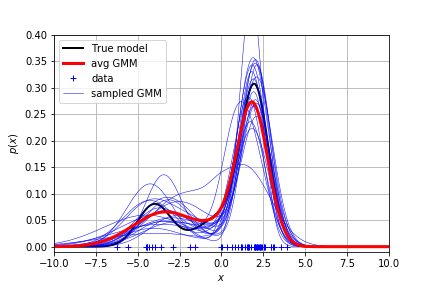## 任务场景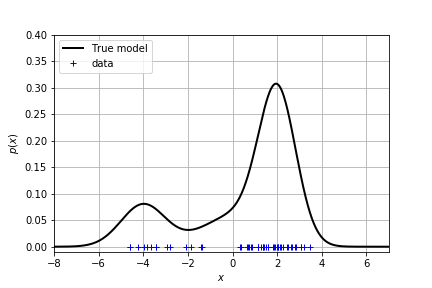## 单峰分布

### 用高斯分布拟合

• $\mu$ is the mean of the Gaussian density
• $\sigma^2$ is the variance of the Gaussian density
• $\lambda = \frac{1}{\sigma^2}$ is the precision of the Gaussian density

（等等我们可以用 $\lambda$ 代替方差来写高斯分布）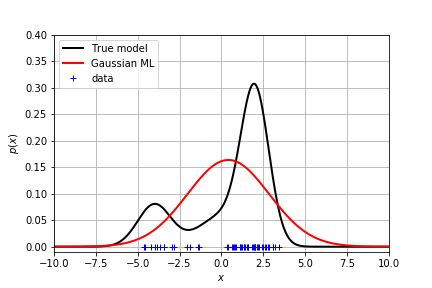### 贝叶斯推理：加入均值和方差的先验

A common and convenient choice of prior for the Gaussian is the Normal-Gamma prior:

where:

$m_0$, $\kappa_0$, $a_0$ and $b_0$ are called hyper-parameters. They are the parameters of the prior distribution.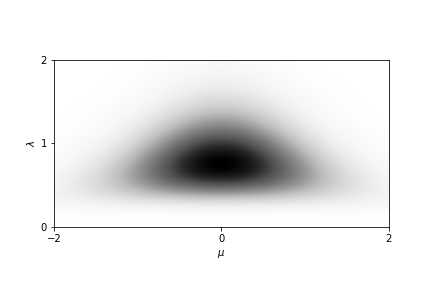where:

$N$ is the total number of point in the the training data and $m_n$, $\kappa_n$, $a_n$ and $b_n$ are the parameters of the posterior. Note that they are different from the hyper-parameters !!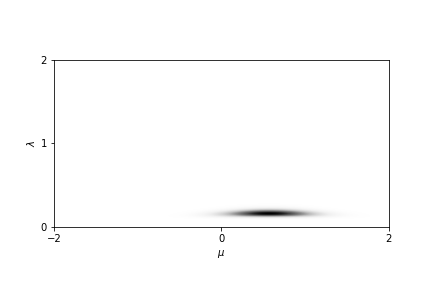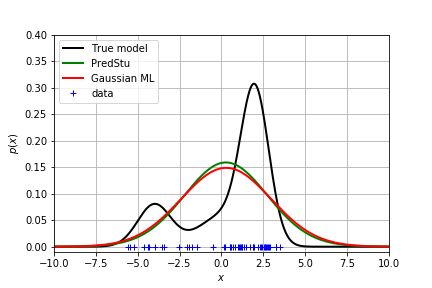## 多峰分布

### 用高斯混合模型拟合

• $\boldsymbol{\mu}$ is the vector of $K$ means
• $\boldsymbol{\lambda}$ is the vector of $K$ precisions
• $\boldsymbol{\pi}$ is the vector of $K$ weights such that $\sum_{k=1}^K \pi_k = 1$

### 用 EM 算法求解参数

• initialize the parameters of the GMM
• iterate until convergence ($\log p(\mathbf{x} | \theta^{new}) - \log p(\mathbf{x} | \theta^{old}) \le 0.01$):
• Expectation (E-step): compute the probability of the latent variable for each data point
• Maximization (M-step): update the parameters from the statistics of the E-step.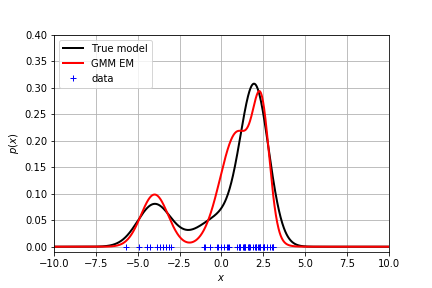### 贝叶斯推理：加入先验

#### mean and variance

We define the set of all data point $x_i$ that are assigned to the component $k$ of the mixture as follows:

and similarly for the latent variables $\mathbf{z}$:

where:

NOTE: these equations are very similar to the Bayesian Gaussian estimate. However, it remains some difference

#### weights

where: